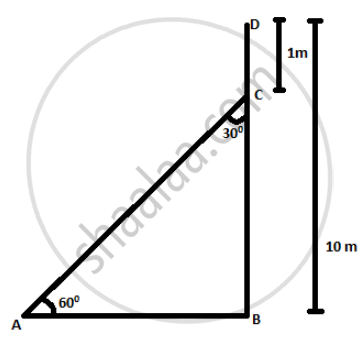# A Technician has to repair light on a pole of height 10 m. She needs to reach a point 1 m below the top of the pole to undertake the repair work. - Mathematics

MCQ

A Technician has to repair light on a pole of height 10 m. She needs to reach a point 1 m below the top of the pole to undertake the repair work. What should be the length of the ladder that she should use which, when inclined at an angle of 60 to the ground, would enable her to reach the required position? Also, how far from the foot of the pole should she place the foot of the ladder?

#### Options

• 1/(6 sqrt3)  "m"

• sqrt3/6  "m"

• 1/sqrt3  "m"

• 6 sqrt3  "m"

#### Solution

6 sqrt3  "m"

Explanation:

The given situation is represented by the figure belowDB – DC = CB

=> "BC" = 9  "m sin" 60^circ = "BCAC" => "BC sin" 60^circ

= 9 sqrt32 = 18 sqrt3 = 6 sqrt3  "m"

Concept: Heights and Distances
Is there an error in this question or solution?